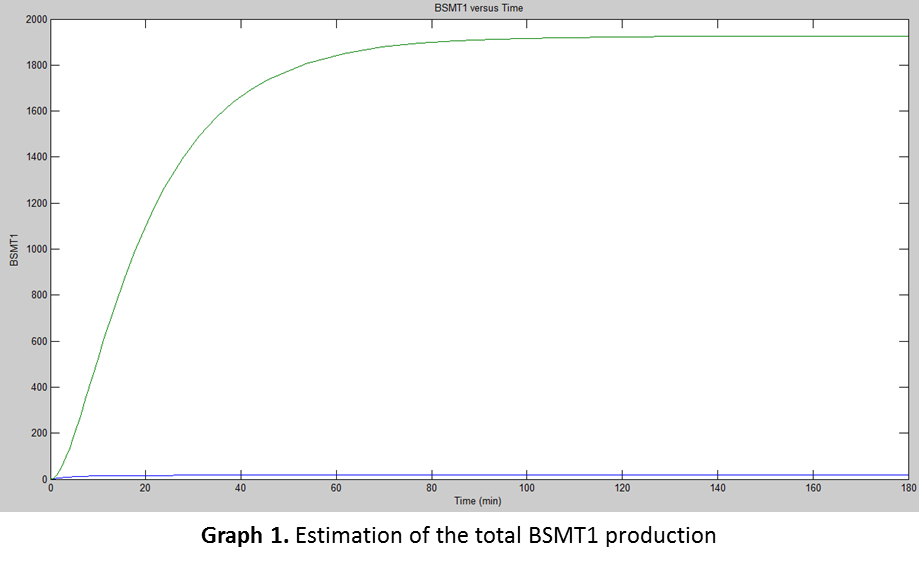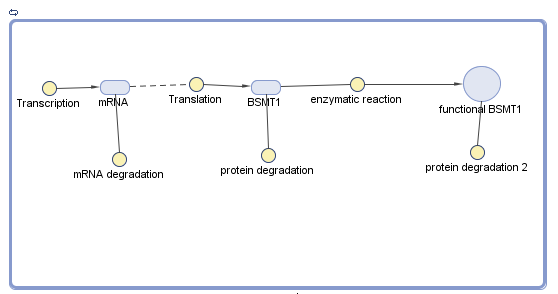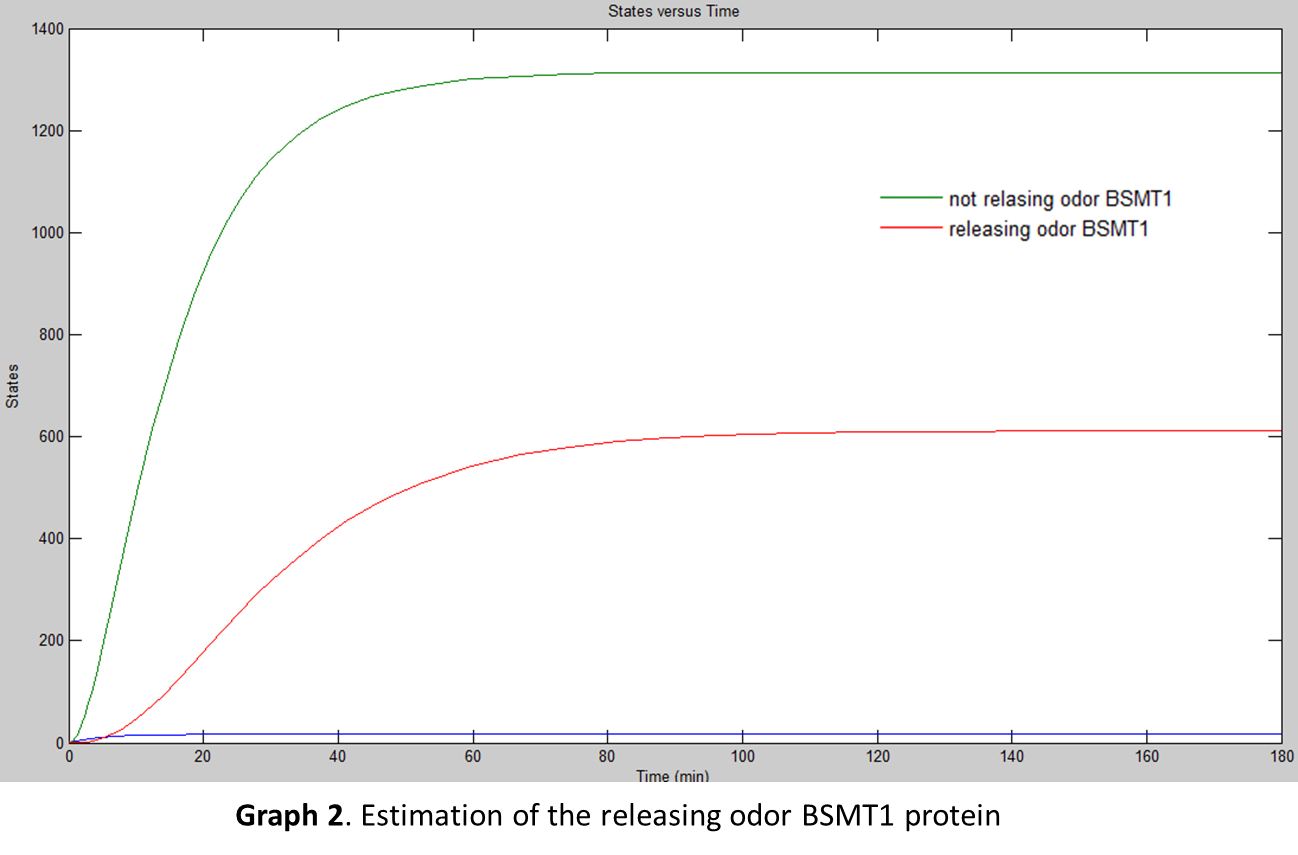# Team:CIDEB-UANL Mexico/math aroma

iGEM CIDEB 2014 - Project

## Aroma Module

The aroma module is based in the production of SAM/salicylic acid methyltransferase (BSMT1opt) in order to generate methyl salicylate, leaving a wintergreen odor. Since this module is not affected by external factors during its transcription, the established formula of mRNA was used.
\begin{equation} \large \frac{d\left [ mRNA \right ]}{dt}=\alpha_{1}-d_{1}\left [ mRNA \right ] \end{equation}

In the case of translation, a RNA thermometer affects the production of the protein BSMT1opt. When the temperature reaches 32°C, the RBS allows the translation of BSMT1 gene, but below 32° C does not. \begin{equation} \large RBS\left\{\begin{matrix} T<32=0 & \\ T\geq 32=1& \end{matrix}\right. \end{equation}
\begin{equation} \large \frac{d[P]}{dt} = \alpha_{2} \cdot[mRNA] - d_{2}[P] - f_{post}\left\{\begin{matrix} T<32 \left\{\begin{matrix} \alpha_{2}=\frac{2400\cdot 0}{358}; RBS=0 & \\ No protein& \end{matrix}\right. & \\ T\geq 32 \left\{\begin{matrix} \alpha_{2}\frac{2400}{358}=6.7& \\ d_{1}\frac{1}{40min}+ \frac{1}{30min}=0.058 & \end{matrix}\right.& \end{matrix}\right. \end{equation}

The parameters for translation and transcription rates from Singapore 2008 iGEM team were used, as well as the transcription and traslation speeds carried out by E. coli, assuming a transcription speed of 70nt/s and a translation speed of 40aa/s. They were used in the equations below with the Wintergreen gene length (1198nt) and protein length (358aa) respectively:

\begin{equation} \large \alpha_{1} = \frac{transcription speed}{gene length (nt)} \end{equation}
\begin{equation} \large \alpha_{2} = \frac{translation speed}{protein length (aa)} \end{equation}
\begin{equation} \large \alpha_{1} = \frac{(70)(60)}{1198} = 3.5 \end{equation}
\begin{equation} \large \alpha_{2} = \frac{(40)(60)}{358} = 6.7 \end{equation}

After, it was needed to use the parameters for degradation rates of proteins and mRNAs obtained from Beijing PKU 2009 iGEM team:

\begin{equation} \large d_{1} = \frac{1}{half-life(min)} + \frac{1}{30min} \end{equation}
\begin{equation} \large d_{2} = \frac{1}{half-life(min)} + \frac{1}{30min} \end{equation}

Since the half-life of BSMT1 has not been determined, a research was made and according to Zubieta (2003), the average half-life for salicylic acid methyltransferases are about 40min.

Later, was used the information from Selinger’s team (2003) to determine the mRNA desgradation. They performed several experiments for finding the average mRNA half-life in E. coli . They used mRNAs of about 1100nt concluding they have an average half-life of 5min. Using the previous relation was found mRNA's half-life from BSMT1opt which was about 5.44min.

\begin{equation} \large HL = \frac{1100(nt)}{5 min} \end{equation}

With the previous data the degradation rates for both, the transcription and the translation of BSMT1opt were found
\begin{equation} \large d_{1} = \frac{1}{5.44} + \frac{1}{30} = 0.21 \end{equation}
\begin{equation} \large d_{2} = \frac{1}{40} + \frac{1}{30} = 0.058 \end{equation}

For the simulation, the team used Simbiology® by plugging in the previously calculated data from the equations to find the amount of proteins E. coli would produce at certain time. The following graph was the result of the simulation:But for the translation, there was another factor taken in consideration, the “fpost, which were the post-translational variables affecting the production of the functional protein.

\begin{equation} \large \frac{d[P]}{dt} = \alpha_{2} \cdot[mRNA] - d_{2}[P] - f_{post} \end{equation}

After researching it was found that BSMT1 is a special type of enzyme called Michaelis-Menten enzyme. BSMT1 reacts with salicylic acid producing methyl salicylate responsible for a Wintergreen odor. As BSMT1 will perform an enzymatic reaction, it was needed to know at which rate it will take place to produce methyl salicylate (Zubieta 2003). For that reason, the post-translational function considers the rate of methyl salicylate production as the variable that directly affects the final protein. The formula is called Michaelis-Menten Equation:

\begin{equation} \large f_{post}=\frac{k_{cat}\left [ S \right ]\left [ E \right ]}{K_{m} + \left [ S \right ]} \end{equation}

Where: “[S]” means the substrate concentration; “[E]”, the enzyme concentration (obtained by the rest of the translational formula); “Kcat; is the turnover number; and “Km, the substrate concentration needed to achieve a half-maximum enzyme velocity.

“Km and “Kcat were established values for SAM (Zublileta, 2003) of 23 and 0.092, respectively. Later, was needed to know the optimal salicylic acid concentration to produce methyl salicylate. Based in the experimental data obtained by our team it was assumed 10mM in 1ml. “[E]” stands for enzyme concentration, or the protein produced. Substituting in the Michaelis-Menten Equation stays as below:

\begin{equation} \large f_{post}=\frac{k_{cat}\left [ S \right ]\left [ E \right ]}{K_{m} + \left [ S \right ]}=\frac{0.092\left ( 10mM \right )\left [ P \right ]}{23 + 10mM}=0.027 \end{equation}

The enzymatic rate was used in Simbiology® to model the amount of BSMT1 which can releases the Wintergreen odor. The results are shown next:When both graphs (Graph 1 and Graph 2) were compared, it was concluded that the enzymatic reaction was too slow compared with the BSMT1 production, leaving only about ½ of releasing odor BSMT1 (about 600) compared with the not releasing odor BSMT1 (about 1300). The BSMT1 unable to release a Wintergreen odor is later degraded leaving a small amount of the functional BSMT1. It was assumed that the functional BSMT1 is the final product, which releases the Wintergreen odor, not too concentrated. It was assumed E. coli would release a slight Wintergreen odor based in the amount of proteins produced.

## Bibliography/References

● DIBDEN, David J. G. (2005). In vivo cycling of the Escherichia coli transcription factor FNR between active and inactive states. Microbiology, 4063-4070.

● SELINGER, Douglas R. M. (2003). Global RNA Half-Life Analysis in Escherichia coli Reveals Positional Patterns of Transcript Degradation. Genome Research, 216-223.

● ZUBIETA, C. (2003). Structural Basis for Substrate Recognition in the Salicylic Acid Carboxyl Methyltransferase Family. Plant Cell, 1704-1716.

iGEM CIDEB 2014 - Footer